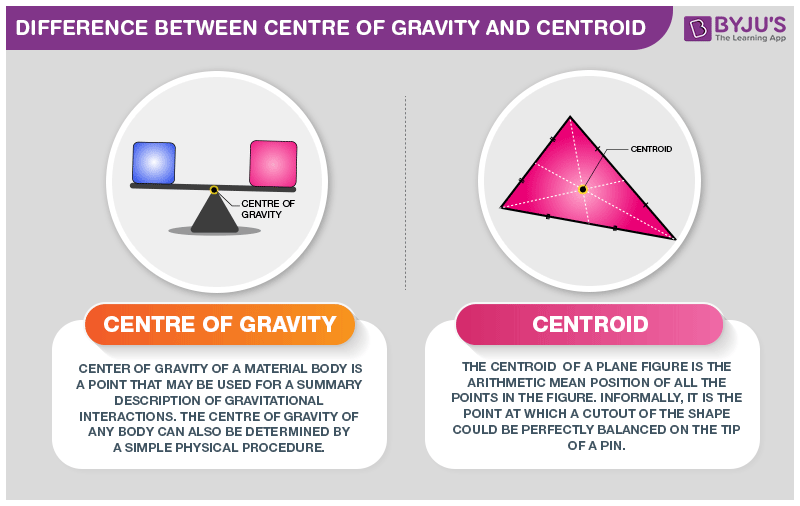# Difference Between Center Of Gravity and Centroid

Every object has some mass and due to gravitational force the object weighs a certain amount. There is a difference between center of gravity and centroid when it is considered with object. These all depends on the factor of uniform or non-uniform distribution of mass across the body.## Difference Between Center Of Gravity and Centroid

In an object, a center of mass is referred to the point where the whole object’s mass is focused that means the point’s mass is represented as the whole object’s mass. The center of gravity of any object is termed to the point where gravity acts on the body.

Where on the other hand, the centroid is referred to the geometrical center of an uniform density object. Which means the object has its weight distributed equally across all parts of the body. If the body is homogeneous (having constant density), then its center of gravity is equivalent with the centroid.

Difference Between Center of Gravity and Centroid

Center of Gravity

Centroid

The point where the total weight of the body focuses upon

It is referred to the geometrical center of a body

It is the point where the gravitational force (weight) acts on the body

It is referred to the center of gravity of uniform density objects

It is denoted by g

It is denoted by c

Center of Gravity in a uniformly gravitational field is the average of all points, weighted by local density or specific weight

The centroid is a point in a plane area in such a way that the moment of area about any axis throughout that point is 0

It is a physical behaviour of the object, a point where all the weight of an object is acting

It is a geometrical behaviour. It is the centre of measure of the amount of geometry.

These were some difference between center of gravity and centroid. If you wish to find out more, download BYJU’S The Learning App.

RELATED ARTICLES: